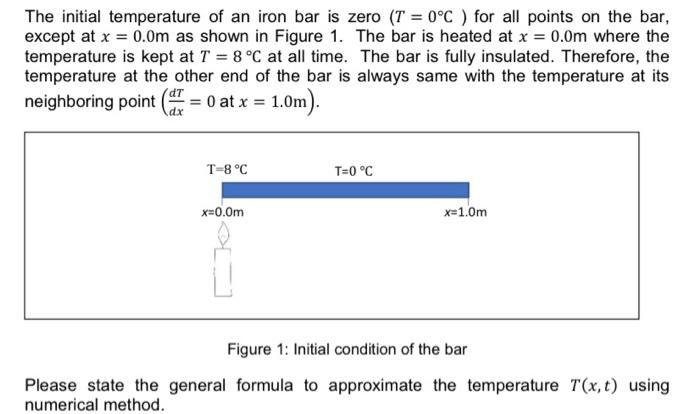# Question formula only The initial temperature of an iron bar is zero (T = 0°C ) for all points on the bar, except at x = 0.0m as shown in Figure 1. The bar is heated at x = 0.0m where the temperature is kept at T = 8 °C at all time. The bar is fully insulated. Therefore, the temperature at the other end of the bar is always same with the temperature at its neighboring point = 0 at x = 1.0m). dx T-8°C T=0 °C x=0.0m x=1.0m Figure 1: Initial condition of the bar Please state the general formula to approximate the temperature T(x,t) using numerical method.RUH1JT The Asker · Mechanical Engineering
formula onlyTranscribed Image Text: The initial temperature of an iron bar is zero (T = 0°C ) for all points on the bar, except at x = 0.0m as shown in Figure 1. The bar is heated at x = 0.0m where the temperature is kept at T = 8 °C at all time. The bar is fully insulated. Therefore, the temperature at the other end of the bar is always same with the temperature at its neighboring point = 0 at x = 1.0m). dx T-8°C T=0 °C x=0.0m x=1.0m Figure 1: Initial condition of the bar Please state the general formula to approximate the temperature T(x,t) using numerical method.
More
Transcribed Image Text: The initial temperature of an iron bar is zero (T = 0°C ) for all points on the bar, except at x = 0.0m as shown in Figure 1. The bar is heated at x = 0.0m where the temperature is kept at T = 8 °C at all time. The bar is fully insulated. Therefore, the temperature at the other end of the bar is always same with the temperature at its neighboring point = 0 at x = 1.0m). dx T-8°C T=0 °C x=0.0m x=1.0m Figure 1: Initial condition of the bar Please state the general formula to approximate the temperature T(x,t) using numerical method.